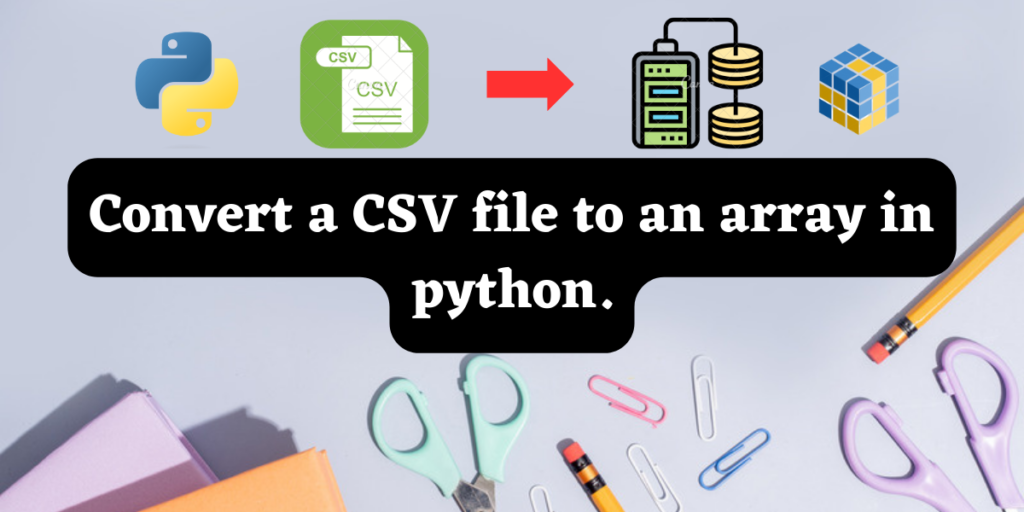# Convert a CSV file to an array in python.In this tutorial, we look at the various methods using which we can convert a CSV file into a NumPy array in Python. CSV files are used to store data values separated by commas. It is useful for database management and used for exchanging or storing in a hassle freeway.

Converting a CSV file into an array allow us to manipulate the data values in a cohesive way and to make necessary changes.

## What is a CSV file?

A plain text file containing data values separated by commas is called a CSV file. CSV stands for Comma Separated Values. The csv format is useful to store data in a tabular manner. It is similar to an excel sheet. Python supports the .csv file format when we import the csv module in our code.

## Need for array creation and using numpy

A CSV file contains huge amounts of data, all of which we might not need during computations. Data scientists and machine learning engineers might need to separate the contains of the CSV file into different groups for successful calculations.

The numerical python or the Numpy library offers a huge range of inbuilt functions to make scientific computations easier. Numpy arrays are data structures that help us to store a range of values. It is n dimensional, meaning its size can be exogenously defined by the user.

Hence we can also easily separate huge CSV files into smaller numpy arrays for ease of computation and manipulation using the functions in the numpy module.

## Prerequisites before we start conversion

Before we jump right into the procedures, we first need to install the following modules in our system. Run the following code in your command prompt in the administrator mode:

```pip install python-csv
pip install numpy
```

Also, you need to have a .csv file in your system which you can use to test out the methods that follow. I have used the file grades.csv in the given examples below.

The file grades.csv has 9 columns and 17-row entries of 17 distinct students in an institute. The 9 columns represent, “last name”, “first name”, and “SSN” (social security number), followed by their scores in 4 different tests and then the final score followed by their grade.

Note: Do not use excel files with .xlsx extension. If you don’t have a .csv version of your required excel file, you can just save it using the .csv extension, or you can use this converter tool.

## Converting a CSV file into a ndarray-2 easy method.

In the first example we will use the np.loadtxt() function and in the second example we will use the np.genfromtxt() function. Both of these functions are a part of the numpy module. Both the functions are extremely easy to use and user friendly. Let’s look at them one by one.

## Method 1- Using the np.loadtxt() function

The following code snippet is very crisp and effective in nature. It is one of the easiest methods of converting a csv file into an array.

```#importing numpy as np
import numpy as np
arr=[] #creating the array
delimiter=",", dtype=str)
print("the array is=")
#displaying our result.
print(arr)
```

The output of the following code will be as shown in the picture below:

## Method 2- Using the numpy genfromtext() method

Another easy method is using the genfromtxt() function from the numpy module. Lets take a look at code involving this method.

```#importing required modules
import numpy as np
#storing all the data in a variable
#constructing the array
Arrd_result = np.genfromtxt(Data, delimiter=",", dtype=str)
print("The array is=")
#displaying the result
print(Arrd_result)
```

The output will be as shown in the image below: